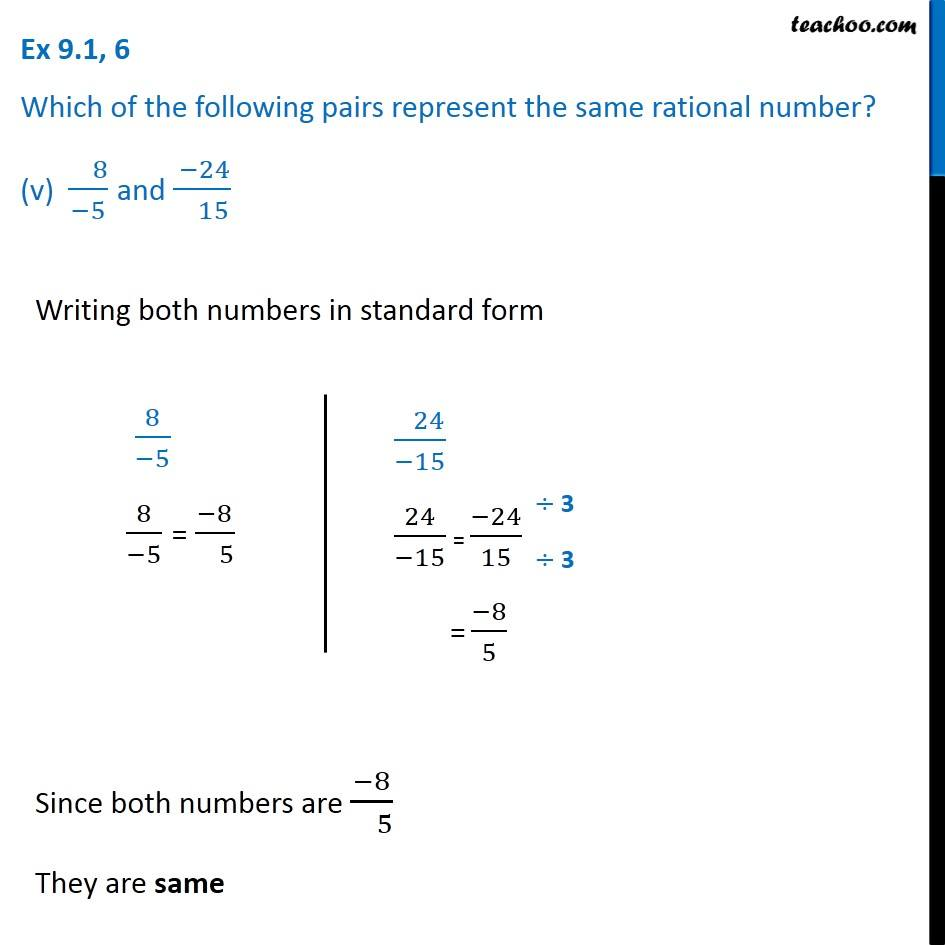Ex 9.1

Chapter 9 Class 7 Rational Numbers
Serial order wiseGet live Maths 1-on-1 Classs - Class 6 to 12

### Transcript

Ex 9.1, 6 Which of the following pairs represent the same rational number? (v) ( 8)/(−5) and ( −24)/( 15) Writing both numbers in standard form 8/(−5) 8/(−5) = (−8)/( 5) ( 24)/(−15) 24/(−15) = (−24)/15 = (−8)/5 Since both numbers are (−8)/( 5) They are same